Скачать презентацию Piecewise and Step Functions It s time to put

5c65f81bad943a1342a17b4e3c3f9859.ppt

• Количество слайдов: 24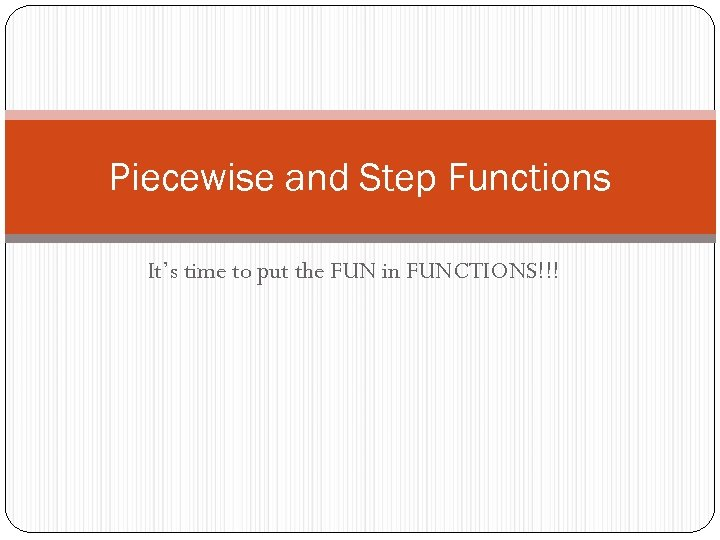Piecewise and Step Functions It’s time to put the FUN in FUNCTIONS!!!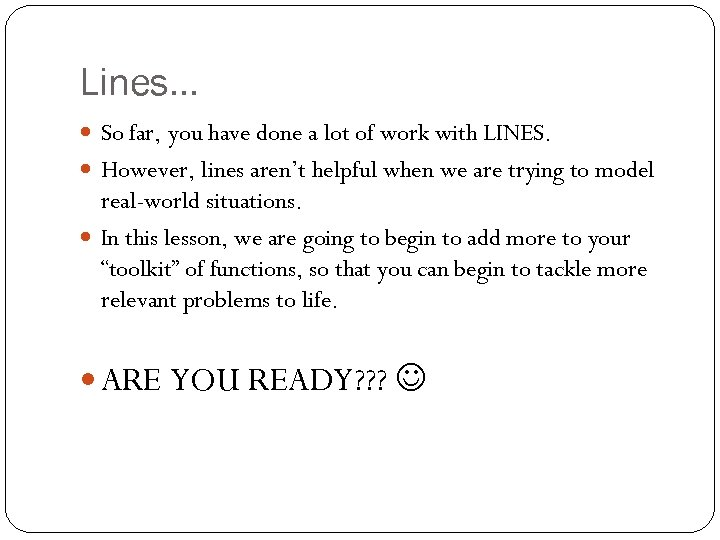Lines… So far, you have done a lot of work with LINES. However, lines aren’t helpful when we are trying to model real-world situations. In this lesson, we are going to begin to add more to your “toolkit” of functions, so that you can begin to tackle more relevant problems to life. ARE YOU READY? ? ?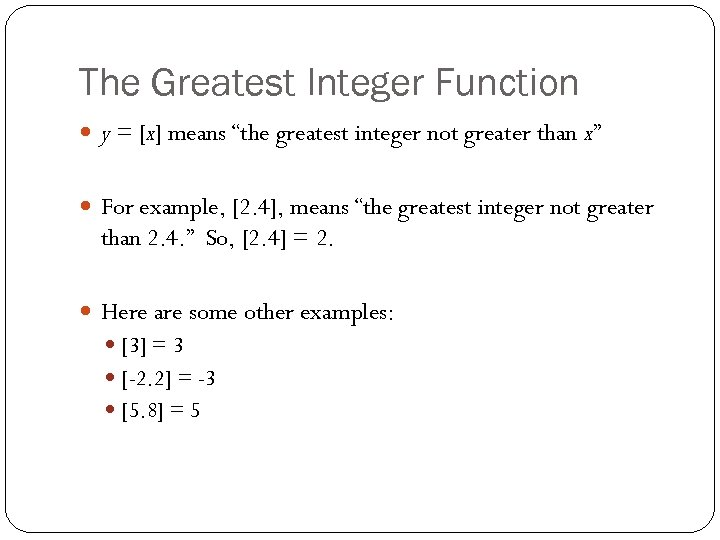The Greatest Integer Function y = [x] means “the greatest integer not greater than x” For example, [2. 4], means “the greatest integer not greater than 2. 4. ” So, [2. 4] = 2. Here are some other examples:  = 3 [-2. 2] = -3 [5. 8] = 5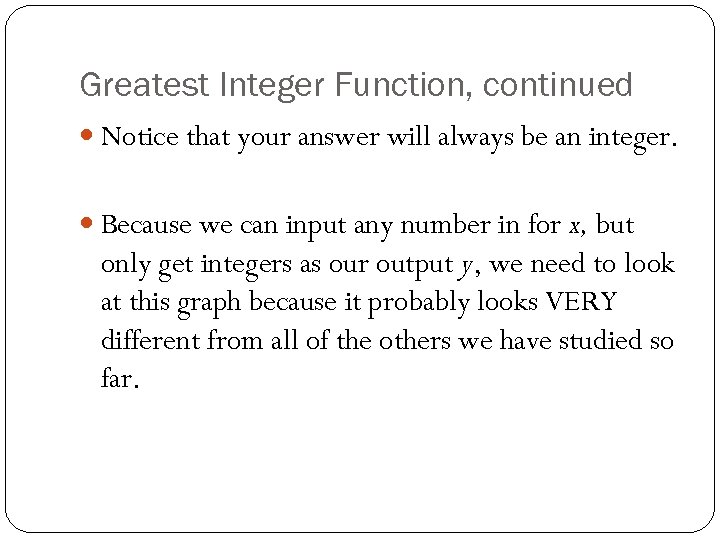Greatest Integer Function, continued Notice that your answer will always be an integer. Because we can input any number in for x, but only get integers as our output y, we need to look at this graph because it probably looks VERY different from all of the others we have studied so far.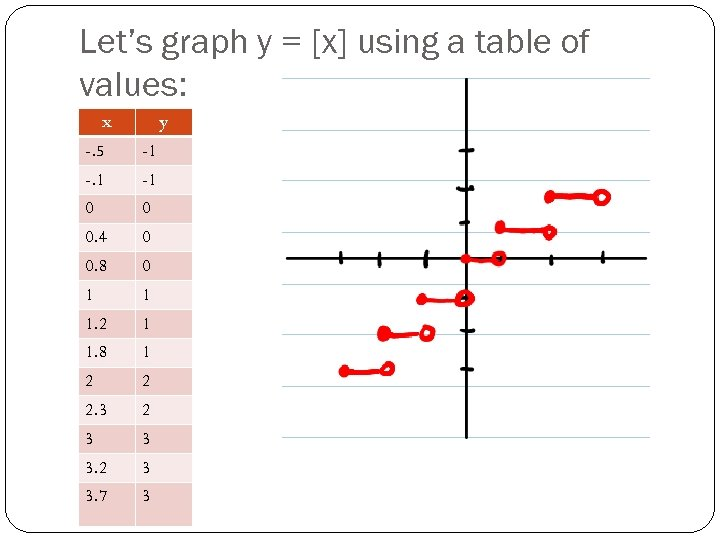Let’s graph y = [x] using a table of values: x y -. 5 -1 -. 1 -1 0 0 0. 4 0 0. 8 0 1 1 1. 2 1 1. 8 1 2 2 2. 3 2 3 3 3. 2 3 3. 7 3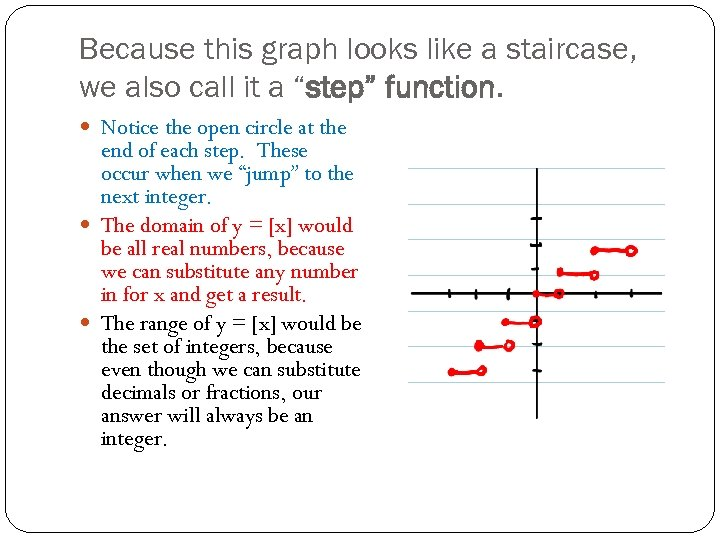Because this graph looks like a staircase, we also call it a “step” function. Notice the open circle at the end of each step. These occur when we “jump” to the next integer. The domain of y = [x] would be all real numbers, because we can substitute any number in for x and get a result. The range of y = [x] would be the set of integers, because even though we can substitute decimals or fractions, our answer will always be an integer.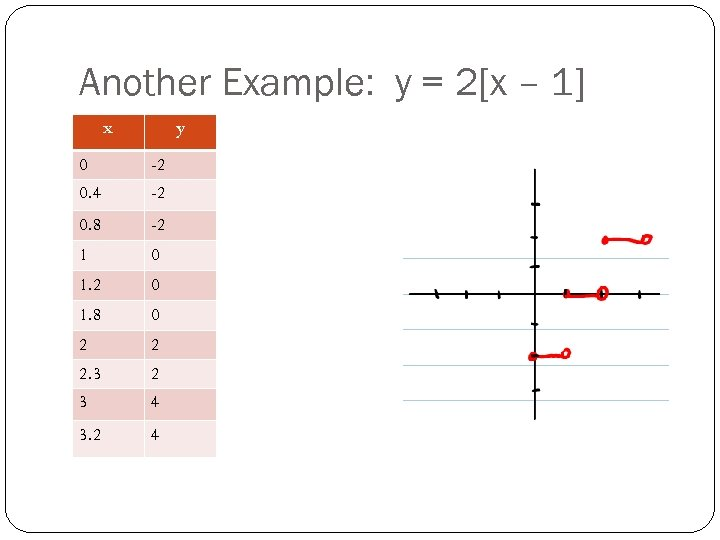Another Example: y = 2[x – 1] x y 0 -2 0. 4 -2 0. 8 -2 1 0 1. 2 0 1. 8 0 2 2 2. 3 2 3 4 3. 2 4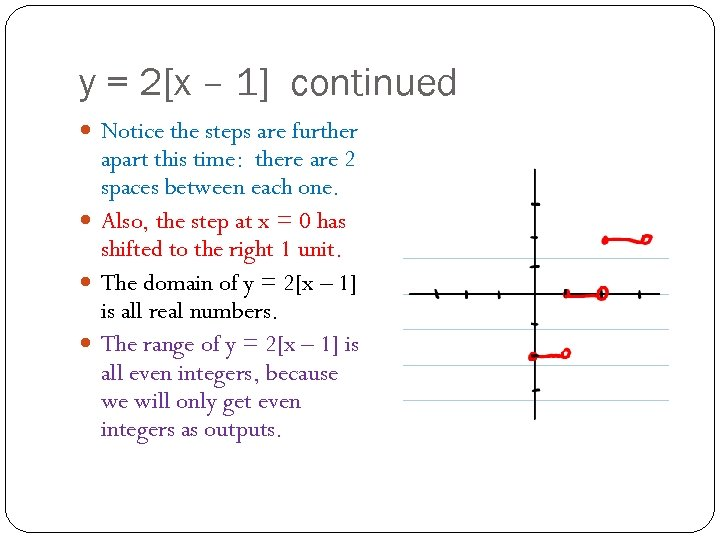y = 2[x – 1] continued Notice the steps are further apart this time: there are 2 spaces between each one. Also, the step at x = 0 has shifted to the right 1 unit. The domain of y = 2[x – 1] is all real numbers. The range of y = 2[x – 1] is all even integers, because we will only get even integers as outputs.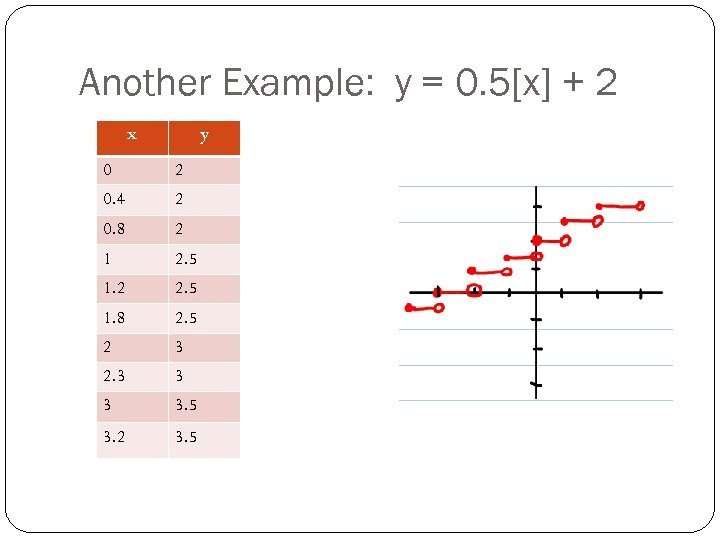Another Example: y = 0. 5[x] + 2 x y 0 2 0. 4 2 0. 8 2 1 2. 5 1. 2 2. 5 1. 8 2. 5 2 3 2. 3 3. 5 3. 2 3. 5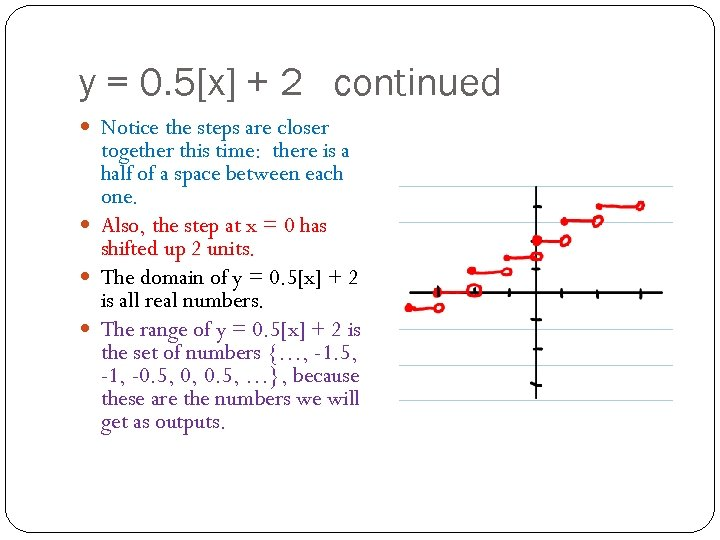y = 0. 5[x] + 2 continued Notice the steps are closer together this time: there is a half of a space between each one. Also, the step at x = 0 has shifted up 2 units. The domain of y = 0. 5[x] + 2 is all real numbers. The range of y = 0. 5[x] + 2 is the set of numbers {…, -1. 5, -1, -0. 5, 0, 0. 5, …}, because these are the numbers we will get as outputs.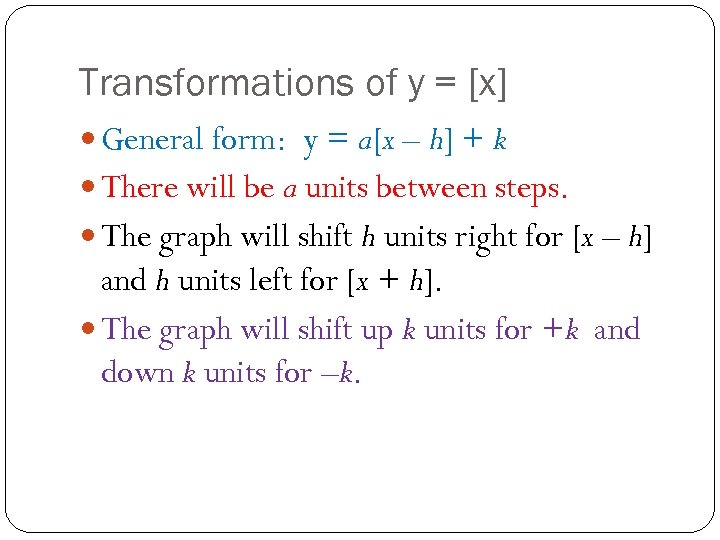Transformations of y = [x] General form: y = a[x – h] + k There will be a units between steps. The graph will shift h units right for [x – h] and h units left for [x + h]. The graph will shift up k units for +k and down k units for –k.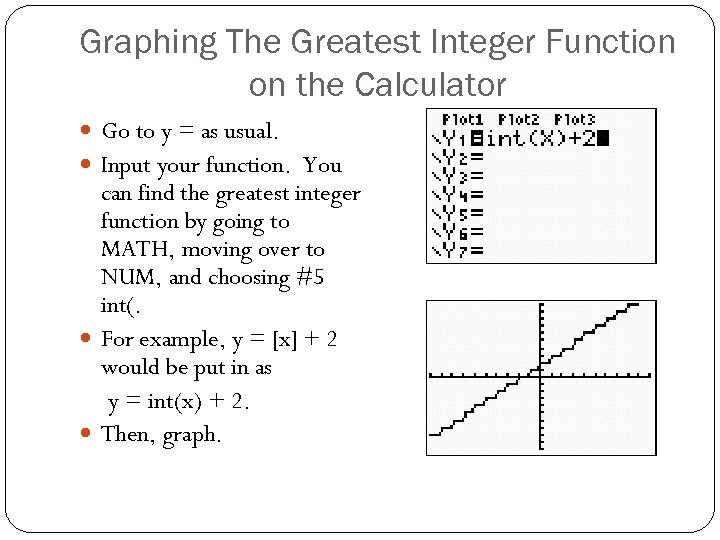Graphing The Greatest Integer Function on the Calculator Go to y = as usual. Input your function. You can find the greatest integer function by going to MATH, moving over to NUM, and choosing #5 int(. For example, y = [x] + 2 would be put in as y = int(x) + 2. Then, graph.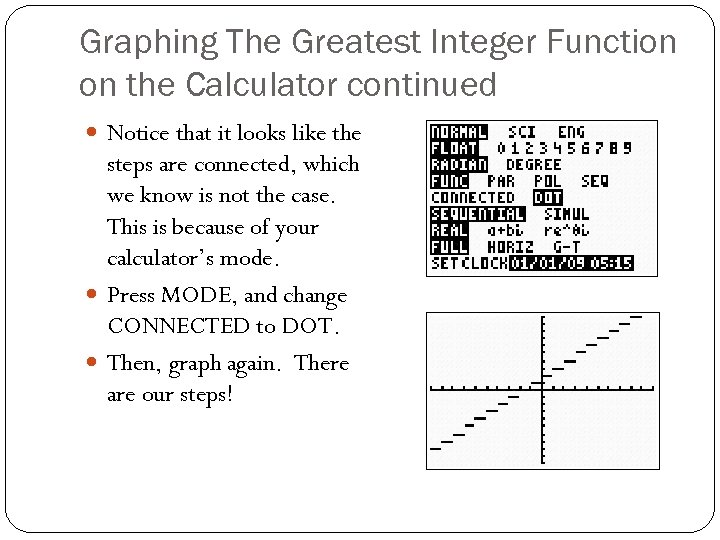Graphing The Greatest Integer Function on the Calculator continued Notice that it looks like the steps are connected, which we know is not the case. This is because of your calculator’s mode. Press MODE, and change CONNECTED to DOT. Then, graph again. There are our steps!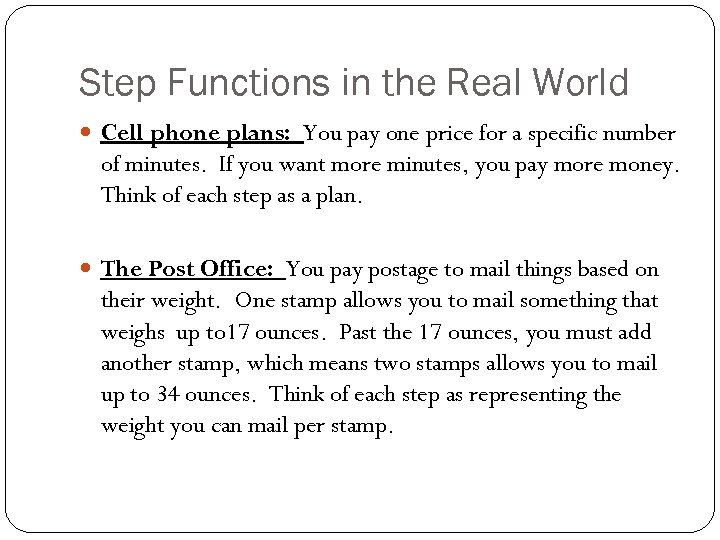Step Functions in the Real World Cell phone plans: You pay one price for a specific number of minutes. If you want more minutes, you pay more money. Think of each step as a plan. The Post Office: You pay postage to mail things based on their weight. One stamp allows you to mail something that weighs up to 17 ounces. Past the 17 ounces, you must add another stamp, which means two stamps allows you to mail up to 34 ounces. Think of each step as representing the weight you can mail per stamp.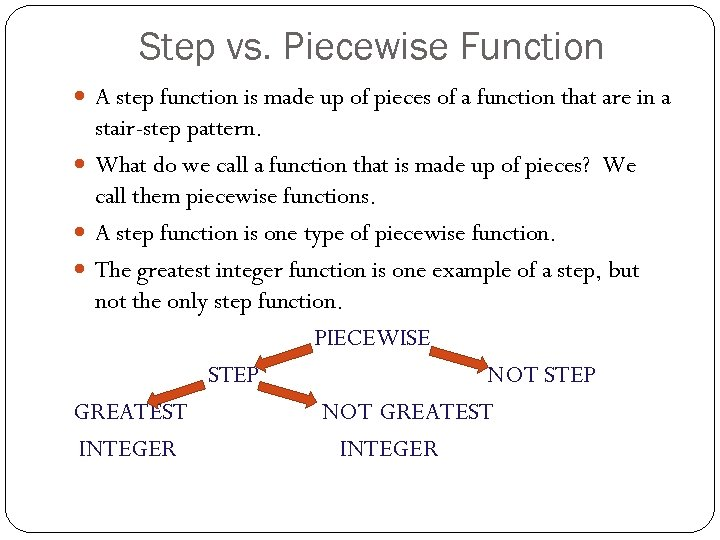Step vs. Piecewise Function A step function is made up of pieces of a function that are in a stair-step pattern. What do we call a function that is made up of pieces? We call them piecewise functions. A step function is one type of piecewise function. The greatest integer function is one example of a step, but not the only step function. PIECEWISE STEP NOT STEP GREATEST NOT GREATEST INTEGER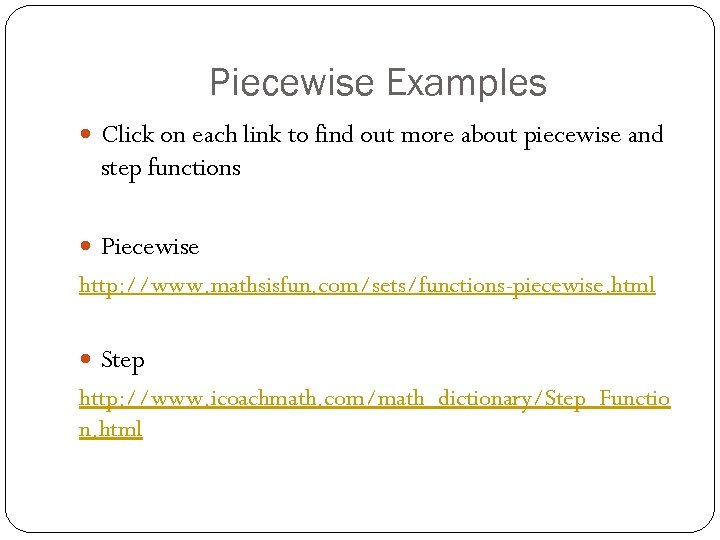Piecewise Examples Click on each link to find out more about piecewise and step functions Piecewise http: //www. mathsisfun. com/sets/functions-piecewise. html Step http: //www. icoachmath. com/math_dictionary/Step_Functio n. html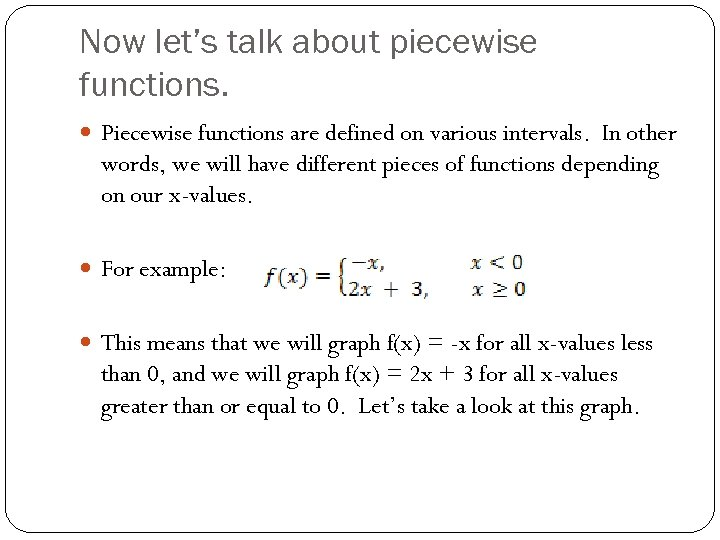Now let’s talk about piecewise functions. Piecewise functions are defined on various intervals. In other words, we will have different pieces of functions depending on our x-values. For example: This means that we will graph f(x) = -x for all x-values less than 0, and we will graph f(x) = 2 x + 3 for all x-values greater than or equal to 0. Let’s take a look at this graph.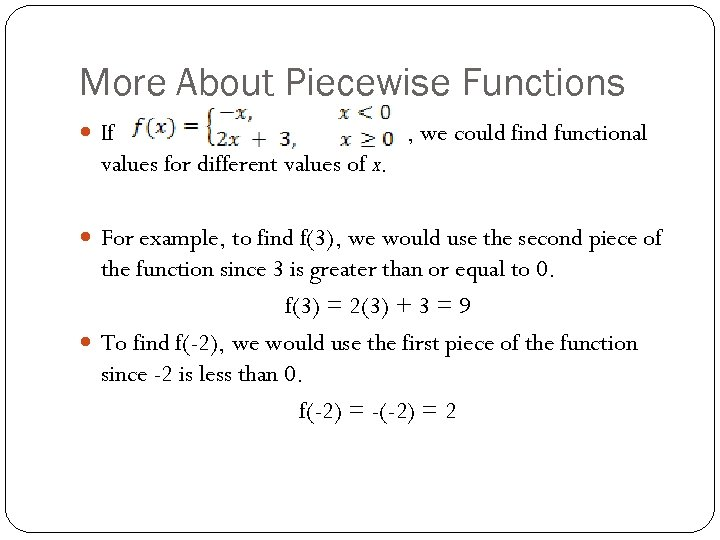More About Piecewise Functions If , we could find functional values for different values of x. For example, to find f(3), we would use the second piece of the function since 3 is greater than or equal to 0. f(3) = 2(3) + 3 = 9 To find f(-2), we would use the first piece of the function since -2 is less than 0. f(-2) = -(-2) = 2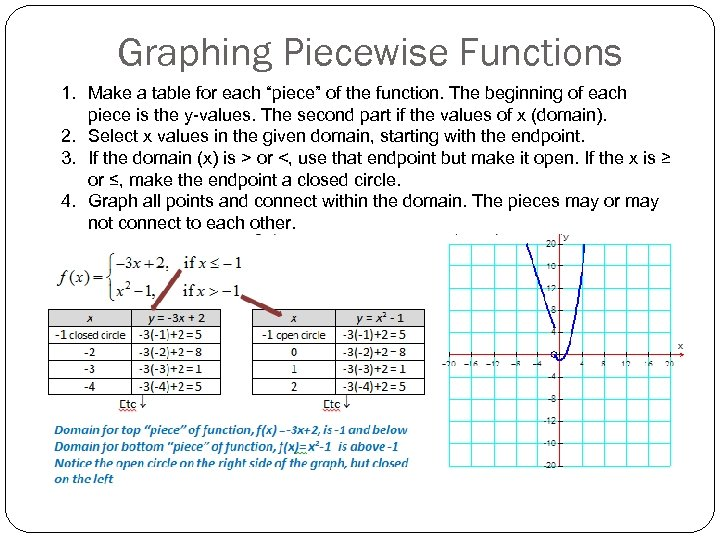Graphing Piecewise Functions 1. Make a table for each “piece” of the function. The beginning of each piece is the y-values. The second part if the values of x (domain). 2. Select x values in the given domain, starting with the endpoint. 3. If the domain (x) is > or <, use that endpoint but make it open. If the x is ≥ or ≤, make the endpoint a closed circle. 4. Graph all points and connect within the domain. The pieces may or may not connect to each other.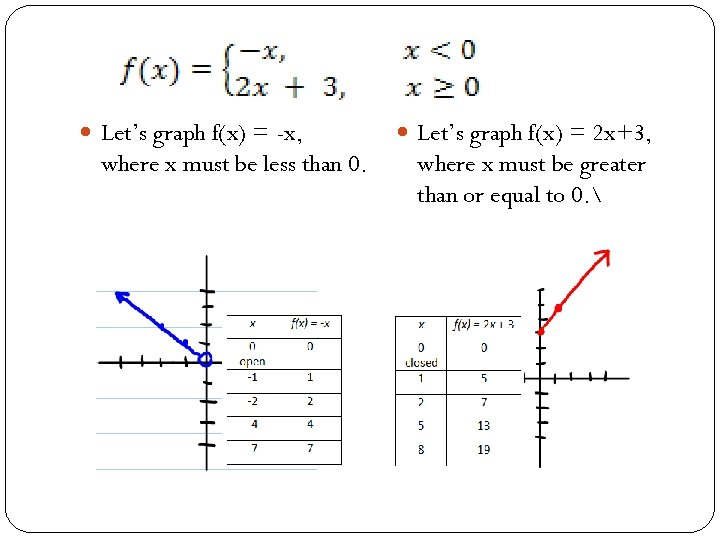Let’s graph f(x) = -x, where x must be less than 0. Let’s graph f(x) = 2 x+3, where x must be greater than or equal to 0.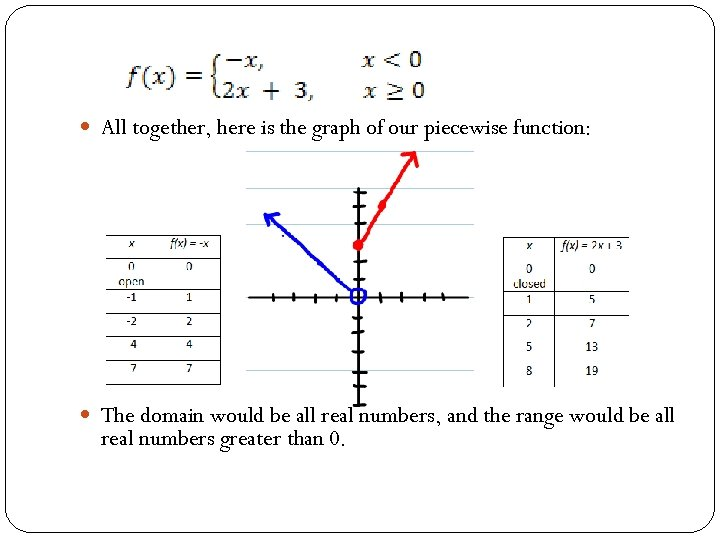All together, here is the graph of our piecewise function: The domain would be all real numbers, and the range would be all real numbers greater than 0.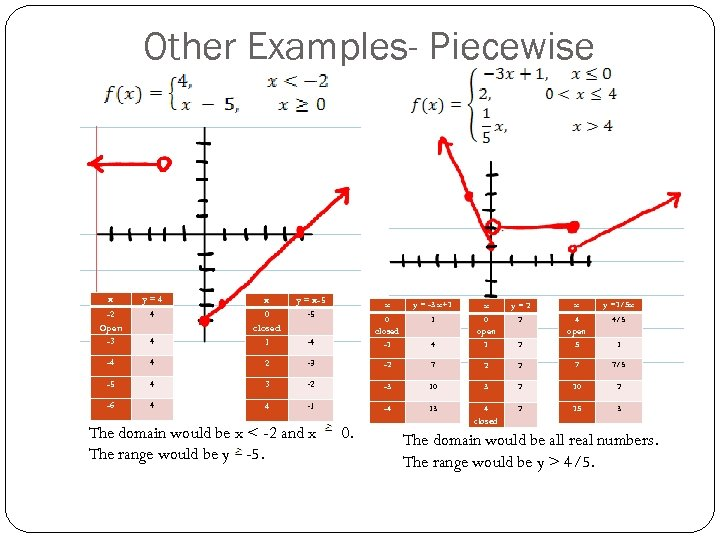Other Examples- Piecewise x -2 Open -3 y=4 4 y = x-5 -5 4 x 0 closed 1 -4 4 -5 -6 x y = -3 x+1 x y=2 x y =1/5 x 1 2 4 open 5 4/5 4 0 open 1 2 -4 0 closed -1 2 -3 -2 7 2 2 7 7/5 4 3 -2 -3 10 3 2 10 2 4 4 -1 -4 13 4 closed 2 15 3 The domain would be x < -2 and x The range would be y -5. 0. 1 The domain would be all real numbers. The range would be y > 4/5.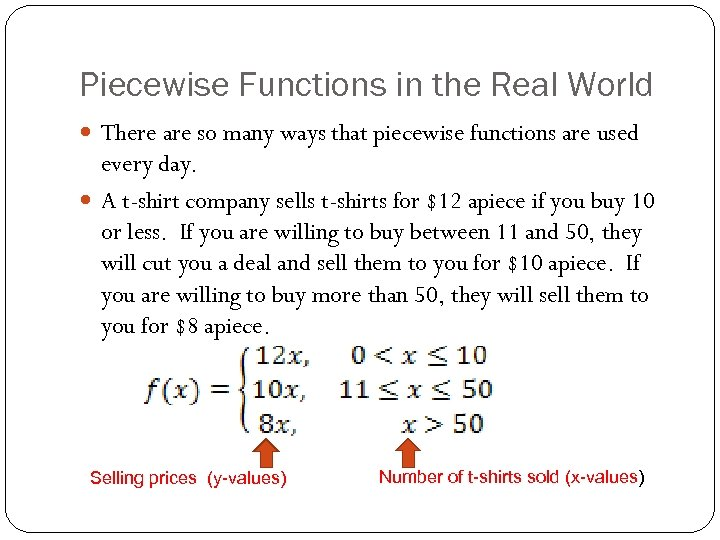Piecewise Functions in the Real World There are so many ways that piecewise functions are used every day. A t-shirt company sells t-shirts for \$12 apiece if you buy 10 or less. If you are willing to buy between 11 and 50, they will cut you a deal and sell them to you for \$10 apiece. If you are willing to buy more than 50, they will sell them to you for \$8 apiece. Selling prices (y-values) Number of t-shirts sold (x-values)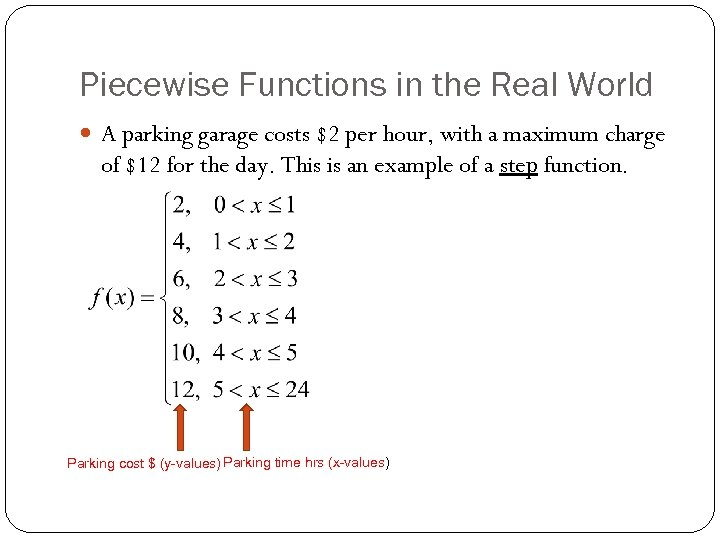Piecewise Functions in the Real World A parking garage costs \$2 per hour, with a maximum charge of \$12 for the day. This is an example of a step function. Parking cost \$ (y-values) Parking time hrs (x-values)